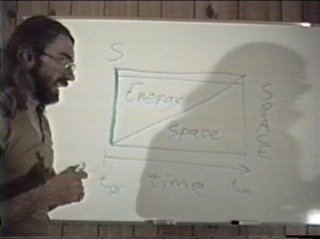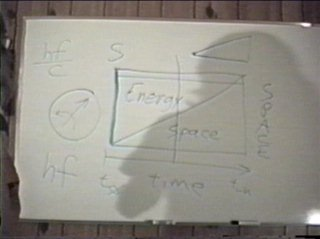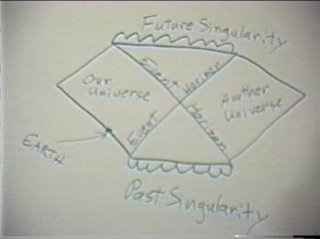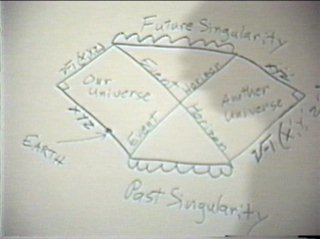# The Relationship Between Energy and SpaceHere I have included lepton space and hadron space in the space-time map. The superposition of the two space-time metrics is required to support lepton structure and the bipolar phenomenon of charge. I re-address this subject many times, it is an integral feature of the string models. At this point though, I simply introduce the duplicitous manifold and ask that it be assumed as an included feature in the subsequent space-time maps.The next space-time map shows the relationship between space and energy in the expanding universe. As the energy-density of space-time drops off, the physical boundaries of space-time increase. It is used to illustrate the translation of energy into space. The only distinction between the quanta's being defined as energy or space is the amount of angular momentum in the quanta. As the cosmological force of charge uses energy (angular momentum) exchanges to redistribute and globally diminish the volume of temporal strings in the manifold, angular momentum is being converted to kinetic thermal energy. (2008 Note: The kinetic component of a quanta's energy makes no contribution to its angular momentum or volume, however in every phase-mixing interaction the quanta participates in where angular momentum is exchanged, there will be a slight reduction of the net angular momentum of the colliding quanta as a product of the recoil or attraction of the quanta. If not for this, the universe would not expand, because there would be nothing to use up the angular momentum and deplete space-time. The horizontal line at the top of the map represents a conserved bit of energy plotted on the map. If a fundamental unit of energy is conserved, it can be drawn as a string in the time-like dimension. Taking a slice out of this fourth-dimensional string to represent how the temporal string appears in conjunction with the phenomenon of the present (now) yields a more or less spherical corpuscle (the shape depends on the "thickness" of the slice of time representing the present. Later I connect the dimensions of the Planck constant as the "slice" of time, which is very thin.) Three geometric attributes are applied to this corpuscle representing a slice of the temporal energy string, a linear component of motion (momentum vector), an axial rotation of the corpuscle about this momentum vector (angular momentum), and a unit volume which is inversely proportional to the energy contained in the angular momentum. Much later in the video I indicate that all energy is angular momentum, and because the moment of angular momentum of a quanta is conserved at +/- h, the scalar energy content of a quanta affects its volume. (Its the only way to keep the moment of angular momentum fixed.) This means that the conceptual tool of "warped space-time" is better thought of as an energy-density gradient in space-time. In the discussion of charge mechanics I show how charge produces such an energy-density gradient around gravitating bodies, and I intimately link charge and gravity as manifestations of an energy exchange the nucleus conducts with space-time. The difference between a quanta and a graviton is in the "handedness" of these two vector quantities. (Like-handed quanta recoil, opposite-handed quanta are attractive.)Kruska's Penrose diagram indicates two universes through the event horizon. I use this diagram (perhaps incorrectly) to support the duplicitous manifold required by this theory. Lepton space and hadron space have a common time-like dimension, but their spatial dimensions are orthogonal (at right angles to each other). In the bottom diagram I have taken the liberty of labeling the dimensions of the various manifolds represented. (This is a hunch, a modified interpretation of Kruska's Penrose diagram.)

Copyright 1994, 2008 by Arnold J. Barzydlo
Go Back Next Page